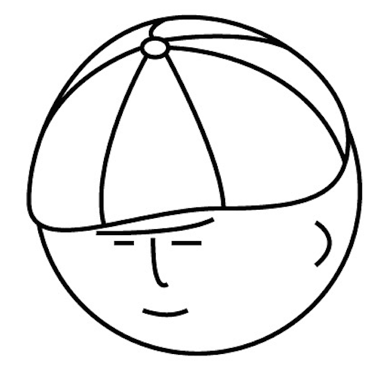# マツシタのお勉強メモ

#### マツシタのお勉強

##### welcome to my engineering blog
###### Hacker Rank# Trie Tree in Java

What is Trie Tree Trie Tree is one of the tree structure to search element. The feature of this tree that each node dose not have element information, but also each edge has element information. Trie tree is used to search String like dict…

# Count Path Sum in Binary Tree

Problem You are given a binary tree in which each node contains an integer value. Find the number of paths that sum to a given value. The path does not need to start or end at the root or a leaf, but it must go downwards (traveling only fr…

# Find the location of the given string from a sorted array of string which is interspersed with empty strings

Problem Given a sorted array of strings which is interspersed with empty strings, write a method to find the location of a given string EXAMPLE Input: find “ball” in {“at”, “”, “”, "ball”, "”, "”, "car”,“”, "”, "dad”, ”“, ”“} Output:4 Solu…

# Print all paths which sum to a given value in a Binary Tree

Problem You are given a binary tree in which each node contains a value. Design an algorithm to print all paths which sum to a given value. The path dose not need to start or end at the root or leaf. Solution First, let’s approach this pro…

# Check if small binary tree T1 is a suibtree of large binary Tree T2

Problem You have two large binary tree: T1, with millions of nodes, and T2, with hundreds of nodes. Create an algorithm to decide if T2 is a subtree of T1. A tree T2 is a subtree of T1 if there exists a node n in T1 such the the subtree of…

# Find the First Common Ancestor of the two nodes in a binary tree

Problem Design an algorithm and write code to find the first common ancestor of two nodes in a binary tree. Avoid storing additional nodes in a data structure. Node: This is not necessarily a binary search tree. Solution I’ll solve this pr…

# Write a method to return all subsets of a set

Problem Write a method to return all subsets of a set Solution This problem can be solve by choosing each element or not. For example we given an array = {1, 2, 3}. First, we do the two patterns that we select 1 or not. By this operation, …

# Find a Magic Index from Sorted Array

Problem A magic index in an array A[] is defined to be an index such that A[i] = i. Given a sorted array of distinct integers, write a method to find a magic index, if one exists, in array A. FOLLOW UP What if the values are not distinct? …

# Robot is moving in grid

Problem Imagine a robot sitting on the upper left corner of an X by Y grid. The robot can only move in two directions: right and down. How many possible paths are there for the robot to go from (0, 0) to (X, Y)? How to Solve By using Dynam…

# Count how many possible ways the child can run up the stairs

Problem A child is running up a staircase with n steps, and can hop either 1 step, 2 steps, or 3 steps at a time. Implement a method to count how many possible ways the child can run up the stairs. How to Solve This problem can be solved e…

# Make a Binary Search Tree from sorted array

Problem Given a sorted (increasing order) array with unique integer elements, write an algorithm to create a binary search tree with minimal height. How to Solve This problem asks me to create a BST (Binary Search Tree) from array. What is…

# Check if a binary tree is balanced

Problem Implement a function to check if a binary tree is balanced. For the purposes of this question. balanced tree is defined to be a tree such that the heights of the two subtrees of any node never differ by more than one. How to Solve …

# Towers of the Hanoi in Java

Problem In the classic problem of the Towers of Hanoi, you have 3 towers and N discs of different sizes which can slide onto any tower. How to Solve First we think about sliding nth disc onto another tower. This handling is composed by the…

# Find the kth to last element of singly Linked List

Problem Implement an algorithm to find the kth to last element of singly Linked List. How to Solve This problem can be solved by Recursive or normal traverse. In recursive case, we have to create IntWrapper Class and implement like this. B…